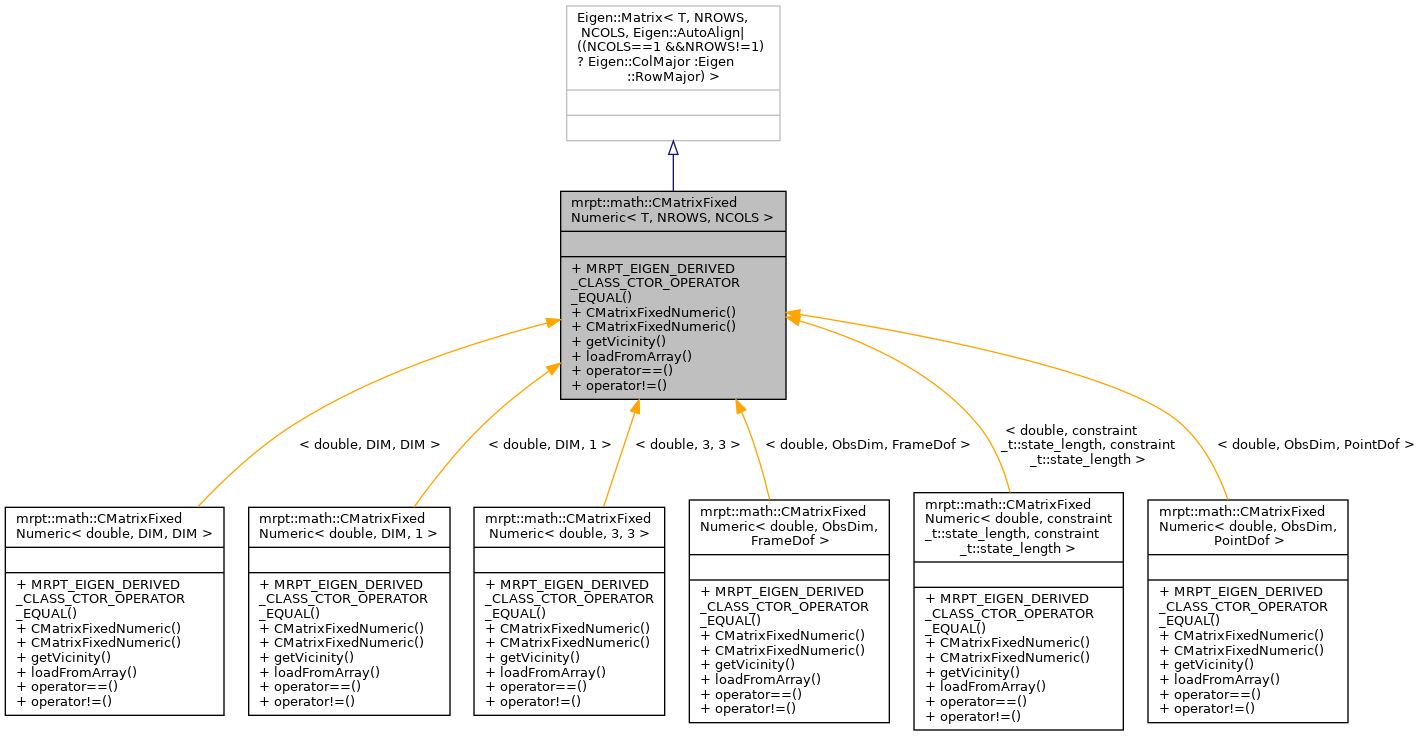Main MRPT website > C++ reference for MRPT 1.5.6
mrpt::math::CMatrixFixedNumeric< T, NROWS, NCOLS > Class Template Reference

Detailed Description

template<typename T, size_t NROWS, size_t NCOLS> class mrpt::math::CMatrixFixedNumeric< T, NROWS, NCOLS >

A numeric matrix of compile-time fixed size.

Basically, this class is a wrapper on Eigen::Matrix<T,NROWS,NCOLS>, but with a RowMajor element memory layout (except for column vectors).

These matrices also have iterators to access all the elements in the matrix as a sequence, starting from the element (0,0), then row by row, from left to right.

Note
This class exists for backward compatibility of ancient times when MRPT didn't rely on Eigen, feel free to directly use Eigen::Matrix<> types instead.
CMatrixTemplateNumeric (for dynamic-size matrices)
Note
For a complete introduction to Matrices and vectors in MRPT, see: http://www.mrpt.org/Matrices_vectors_arrays_and_Linear_Algebra_MRPT_and_Eigen_classes

Definition at line 34 of file CMatrixFixedNumeric.h.

`#include <mrpt/math/CMatrixFixedNumeric.h>`

Inheritance diagram for mrpt::math::CMatrixFixedNumeric< T, NROWS, NCOLS >:Public Types

typedef Eigen::Matrix< T,
NROWS, NCOLS, Eigen::AutoAlign|((NCOLS==1
&&NROWS!=1)?Eigen::ColMajor:Eigen::RowMajor) >
Base

typedef CMatrixFixedNumeric< T,
NROWS, NCOLS >
mrpt_autotype

Public Member Functions

MRPT_EIGEN_DERIVED_CLASS_CTOR_OPERATOR_EQUAL (CMatrixFixedNumeric) inline CMatrixFixedNumeric()
Default constructor, initializes all elements to zero. More...

CMatrixFixedNumeric (const T *vals)
Constructor from an array in row major. More...

CMatrixFixedNumeric (TConstructorFlags_Matrices)
Constructor which leaves the matrix uninitialized. More...

template<size_t N, typename ReturnType >
ReturnType getVicinity (size_t c, size_t r) const

template<typename Derived >
bool operator== (const Eigen::MatrixBase< Derived > &m2) const
== comparison of two matrices; it differs from default Eigen operator in that returns false if matrices are of different sizes instead of raising an assert. More...

template<typename Derived >
bool operator!= (const Eigen::MatrixBase< Derived > &m2) const
!= comparison of two matrices; it differs from default Eigen operator in that returns true if matrices are of different sizes instead of raising an assert. More...

Member Typedef Documentation

template<typename T, size_t NROWS, size_t NCOLS>
 typedef Eigen::Matrix mrpt::math::CMatrixFixedNumeric< T, NROWS, NCOLS >::Base

Definition at line 45 of file CMatrixFixedNumeric.h.

template<typename T, size_t NROWS, size_t NCOLS>
 typedef CMatrixFixedNumeric mrpt::math::CMatrixFixedNumeric< T, NROWS, NCOLS >::mrpt_autotype

Definition at line 46 of file CMatrixFixedNumeric.h.

Constructor & Destructor Documentation

template<typename T, size_t NROWS, size_t NCOLS>
 mrpt::math::CMatrixFixedNumeric< T, NROWS, NCOLS >::CMatrixFixedNumeric ( const T * vals )
inline

Constructor from an array in row major.

Definition at line 55 of file CMatrixFixedNumeric.h.

template<typename T, size_t NROWS, size_t NCOLS>
 mrpt::math::CMatrixFixedNumeric< T, NROWS, NCOLS >::CMatrixFixedNumeric ( TConstructorFlags_Matrices )
inline

Constructor which leaves the matrix uninitialized.

Example of usage: CMatrixFixedNumeric<double,3,2> M(mrpt::math::UNINITIALIZED_MATRIX);

Definition at line 60 of file CMatrixFixedNumeric.h.

Member Function Documentation

template<typename T, size_t NROWS, size_t NCOLS>
template<size_t N, typename ReturnType >
 ReturnType mrpt::math::CMatrixFixedNumeric< T, NROWS, NCOLS >::getVicinity ( size_t c, size_t r ) const
inline

Definition at line 62 of file CMatrixFixedNumeric.h.

template<typename T, size_t NROWS, size_t NCOLS>
 void mrpt::math::CMatrixFixedNumeric< T, NROWS, NCOLS >::loadFromArray ( const T * vals )
inline
template<typename T, size_t NROWS, size_t NCOLS>
 mrpt::math::CMatrixFixedNumeric< T, NROWS, NCOLS >::MRPT_EIGEN_DERIVED_CLASS_CTOR_OPERATOR_EQUAL ( CMatrixFixedNumeric< T, NROWS, NCOLS > )
inline

Default constructor, initializes all elements to zero.

Definition at line 48 of file CMatrixFixedNumeric.h.

template<typename T, size_t NROWS, size_t NCOLS>
template<typename Derived >
 bool mrpt::math::CMatrixFixedNumeric< T, NROWS, NCOLS >::operator!= ( const Eigen::MatrixBase< Derived > & m2 ) const
inline

!= comparison of two matrices; it differs from default Eigen operator in that returns true if matrices are of different sizes instead of raising an assert.

Definition at line 82 of file CMatrixFixedNumeric.h.

template<typename T, size_t NROWS, size_t NCOLS>
template<typename Derived >
 bool mrpt::math::CMatrixFixedNumeric< T, NROWS, NCOLS >::operator== ( const Eigen::MatrixBase< Derived > & m2 ) const
inline

== comparison of two matrices; it differs from default Eigen operator in that returns false if matrices are of different sizes instead of raising an assert.

Definition at line 74 of file CMatrixFixedNumeric.h.

 Page generated by Doxygen 1.8.6 for MRPT 1.5.6 Git: 4c65e84 Tue Apr 24 08:18:17 2018 +0200 at mar abr 24 08:26:17 CEST 2018